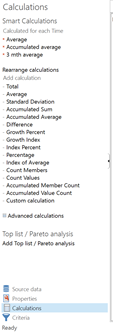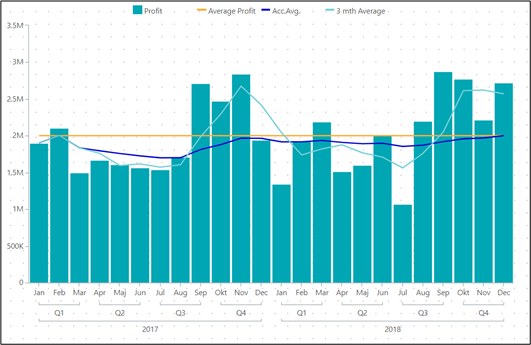# Calculations in graphs

Let’ s look into how to use reference ranges and relative references.

• Add a Column chart to the Profit Analysis, Profit per Time YMD (on month level).
• Add the following calculations to the Column chart (all as a single column):
• Average = avg(d-1, all, m1) (demonstrating the all reference range)
• Accumulated average = avg(d-1, d1:0, m1) (demonstrating an absolute reference range)
• 3 mth average = avg(d-1, -2:0, m1) (demonstrating a relative reference)
• Change visualization for each of the three calculations to a Line.
• The final result should now look something like this: